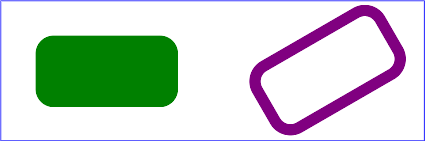The shape methods

Shape methods

The shape methods listed below are defined in the RVG, RVG::ClipPath, RVG::Group, and RVG::Pattern classes. You can chain the styles method and the transform methods to the shape methods.

shape

circle

Description

Adds a circle to the target object. The arc of a circle begins at the "3 o'clock" point on the radius and progresses towards the "9 o'clock" point. The starting point and direction of the arc are affected by the user space transform in the same manner as the geometry of the object.

Arguments
cx, cy
The center of the circle
Example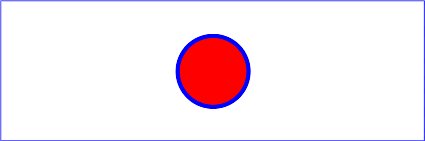ellipse

obj.ellipse(rx, ry, cx=0, cy=0) -> ellipse

Description

Adds an ellipse to the target object. The arc of an ellipse begins at the "3 o'clock" point on the radius and progresses towards the "9 o'clock" point. The starting point and direction of the arc are affected by the user space transform in the same manner as the geometry of the object.

Arguments
rx, ry
The x- and y-radii of the ellipse
cx, cy
The center of the ellipse
Exampleline

obj.line(x1=0, y1=0, x2=0, y2=0) -> line

Description

Adds a line to the target object. Lines are never filled.

Arguments
x1, y1
The starting point of the line
x2, y2
The ending point of the line
Examplepath

obj.path(path_data) -> path

Description

Adds a path to the target object.

Arguments

A path string. The path string has the same syntax as the d= attribute on SVG's path element. See the SVG standard for a complete description of the syntax.

Examples

moveto, lineto, and closepath commands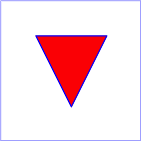simple uses of cubic Bézier commands within a path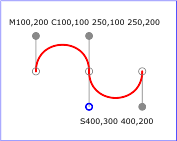cubic Bézier commands change their shape according to the position of the control points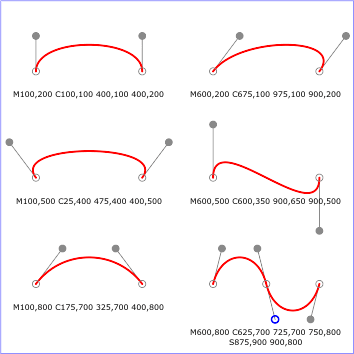simple uses of quadratic Bézier commands within a pathsimple uses of arc commands within a path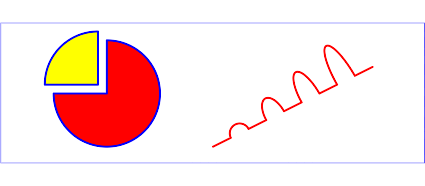Elliptical arcs: The following illustrates the four combinations of large-arc-flag and sweep-flag and the four different arcs that will be drawn based on the values of these flags.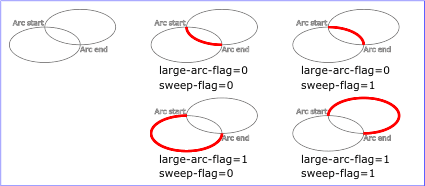polygon

obj.polygon(x1, y1, x2, y2...) -> polygon
obj.polygon(array) -> polygon
obj.polygon(array1, array2) -> polygon

Description

Adds a closed shape consisting of a series of connected line segments to the target object.

Arguments

The arguments to polygon and polyline can be

1. At least 4 numbers that describe the [x, y] coordinates of the points of the polygon/polyline.
2. One array containing at least 4 numbers.
3. Two arrays. The first array is a list of x-coordinates. The second array is a list of y-coordinates. Both arrays must have at least one element. If one array is shorter than the other, the shorter array is extended by duplicating its elements as necessary. The combined arrays must describe at least 2 pairs of [x,y] coordinates. For example
x = [1, 3, 5, 7, 9]
y = [2,4]
canvas.polygon(x, y)
# is equivalent to canvas.polygon(1,2, 3,4, 5,2, 7,4, 9,2)

It is an error to specify an odd number of coordinates. Array arguments can be any objects that can be converted to arrays by the Kernel#Array method.

Example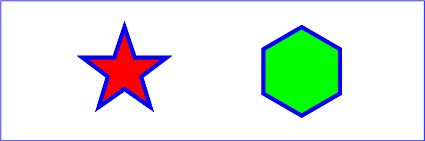polyline

obj.polyline(x1, y1, x2, y2...) -> polyline
obj.polyline(array) -> polyline
obj.polyline(array1, array2) -> polyline

Description

Adds a set of connected lines segments to the target object. Typically a polyline defines an open shape.

See polygon

Example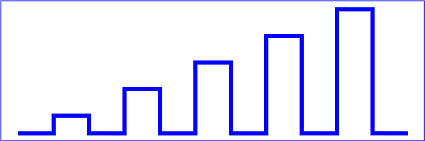rect

obj.rect(width, height, x=0, y=0) -> rect

Description

Adds a rectangle to the target object.

Arguments
width, height
The width and height of the rectangle
x, y
The x- and y-axis coordinates of the upper-left corner
Example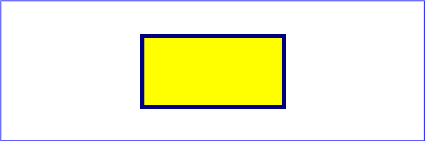Rounded rectangles

You can define a rounded rectangle by chaining the round method to rect:

obj.rect(width, height, x=0, y=0).round(rx[, ry])

The round method accepts two arguments.

rx
The x-axis radius of the ellipse used to round off the corners of the rectangle
ry
The y-axis radius of the ellipse used to round off the corners of the rectangle

If the second argument is omitted it defaults to the value of the first argument.

Example